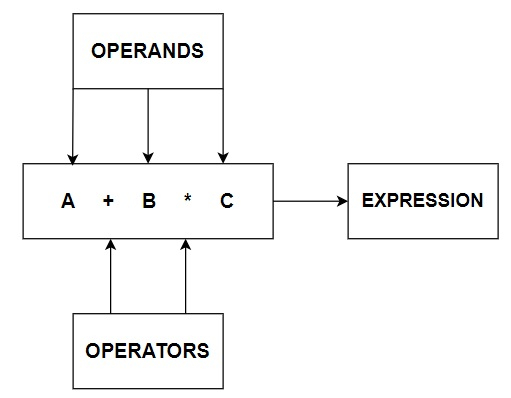# What are the bitwise operators in Java?

## OPERATORS

In computer programming we often need to perform some arithmetical or logical operations. In such circumstances, we need operators to perform these tasks. Thus, an Operator is basically a symbol or token, which performs arithmetical or logical operations and gives us meaningful result. The values involved in the operation are called Operands.

Here is a basic Pictorial representation of Operators.## BITWISE OPERATORS

In Java Programming, you can use some special types of operators, which perform operations on bit level of the operands. These operators use byte, short int and long type operands. However, float and double types are not allowed. Bitwise operators are one type of binary operators.

OPERATORS MEANING
& Bitwise AND
| Bitwise OR
^ Bitwise XOR
! Bitwise NOT
~ Bitwise complement
<< Left shift
>> Right shift
>>> Zero fill right shift
<<= Left shift assignment
>>= Right shift assignment
>>>= Zero fill right shift assignment

Let’s talk about each of the operators in details.

Bitwise AND

This operator results in high (1) if both the operands are high, otherwise low (0).

Truth Table of AND operation

a b a & b
0 0 0
0 1 0
1 0 0
1 1 1

Bitwise OR

This operator results in high (1), if either of two operands is high, otherwise low (0).

Truth Table of OR operation

a b a | b
0 0 0
0 1 1
1 0 1
1 1 1

Bitwise XOR

This operator results in low (0) for the same values of the operands. The outcome is high (1) for the different values.

Truth Table of XOR operation

a b a ^ b
0 0 0
0 1 1
1 0 1
1 1 0

Bitwise NOT

This operator results in high (1) if bit operand is low (0) and vice versa. Bitwise NOT is a unary operator.

Truth Table of NOT operation

a !a
0 1
1 0

Bitwise Complement

This operator results in high (1) if bit operand is low (0) and vice versa. Bitwise Complement is a unary operator.

Truth Table of Complement operation

a ~a
0 1
1 0

Left Shift

This operator shifts all bits towards the left by a certain number of specified bits.

For Example − We are going to perform a single Left shift on 1011.

Step 1 − Before Left Shift

 1 0 1 1

Step 2 − After Left Shift

 1 0 1 1 0

The rightmost bit is the replacement bit, whereas the leftmost bit is the discarded bit.

So, the new number is 0110.

Right Shift

This operator shifts all bits towards the right by a certain number of specified bits.

For Example − We are going to perform a single Right shift on 1011.

Step 1 − Before Right Shift

 1 0 1 1

Step 2 − After Right Shift

 0 1 0 1 1

The rightmost bit is the discarded bit, whereas the leftmost bit is the replacement bit.

So, the new number is 0101.

Zero fill right shift

This operator shifts all bits towards the right by a certain number of specified bits. The difference between Right Shift and Zero fill Right Shift is Right Shift preserves the sign bit (i.e. if a = -8, then a >> 2 will be a negative number) whereas Zero fill Right Shift doesn’t consider the sign bit (i.e. if a = -8, then a >>> 2 will be a positive number).

For Example − We are going to perform a Zero fill Right shift on 1011.

Step 1 − Before Right Shift

 1 0 1 1

Step 2 − After Right Shift

 0 1 0 1 1

The rightmost bit is the discarded bit, whereas the leftmost bit is the replacement bit.

So, the new number is 0101.

Left shift assignment

This operator left shifts the left operand by number of positions given by the right operand.

For Example − Let us assume a = 5, binary value of 5 is 00101, Hence a <<= 2 will give the binary value 10100 which is 20.

Right shift assignment

This operator right shifts the left operand by number of positions given by the right operand.

For Example − Let us assume a = 5, binary value of 5 is 00101, Hence a >>= 2 will give the binary value 00001 which is 1.

Let us assume b = -5, binary value of -5 is -00101, Hence a >>= 2 will give the binary value -10 (for signed numbers, the sign bit is used to fill the vacated bit positions i.e. if the number > 0, 0 is used, and if < 0 then 1 is used.) which is -2.

Zero fill right shift assignment

This operator right shifts the left operand by number of positions given by the right operand. What’s different in this case is if we zero fill right shift e negative number it will return a positive integer.

For Example: Let us assume a = 5, binary value of 5 is 00101, Hence a >>>= 2 will give the binary value 00001 which is 1.

Let us assume b = -5, binary value of -5 is 11111111111111111111111111111011, Hence b >>>= 2 will give the binary value 00111111111111111111111111111110, which is 1073741822.